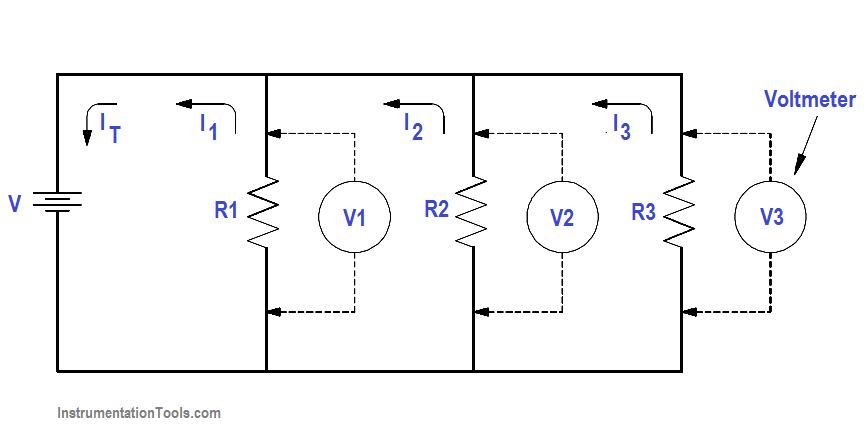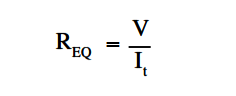# Parallel Circuit

Parallel circuits are those circuits which have two or more components connected across the same voltage source (Figure 17). Resistors R 1 ,R2, and R3 are in parallel with each other and the source.

Each parallel path is a branch with its own individual current. When the current leaves the source V, part I1 of IT will flow through R1; part I2 will flow through R2 ; and part I3 will flow through R3 . Current through each branch can be different; however, voltage throughout the circuit will be equal.

##### V = V1 = V2 = V3Figure 17 Parallel Circuit

#### Equivalent Resistance

In a parallel circuit, the total resistance of the resistors in parallel is referred to as equivalent resistance. This can be described as the total circuit resistance as seen by the voltage source. In all cases, the equivalent resistance will be less than any of the individual parallel circuit resistors.

Using Ohm’s Law, equivalent resistance (REQ ) can be found by dividing the source voltage (V) by the total circuit current (IT ), as shown in Figure 17.